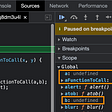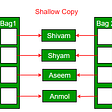# Solving in JS. FrogRiverOne

## Question.

`A small frog wants to get to the other side of a river. The frog is initially located on one bank of the river (position 0) and wants to get to the opposite bank (position X+1). Leaves fall from a tree onto the surface of the river.You are given an array A consisting of N integers representing the falling leaves. A[K] represents the position where one leaf falls at time K, measured in seconds.The goal is to find the earliest time when the frog can jump to the other side of the river. The frog can cross only when leaves appear at every position across the river from 1 to X (that is, we want to find the earliest moment when all the positions from 1 to X are covered by leaves). You may assume that the speed of the current in the river is negligibly small, i.e. the leaves do not change their positions once they fall in the river.For example, you are given integer X = 5 and array A such that:A = 1   A = 3   A = 1   A = 4   A = 2   A = 3   A = 5   A = 4In second 6, a leaf falls into position 5. This is the earliest time when leaves appear in every position across the river.Write a function:that, given a non-empty array A consisting of N integers and integer X, returns the earliest time when the frog can jump to the other side of the river.If the frog is never able to jump to the other side of the river, the function should return −1.For example, given X = 5 and array A such that:A = 1   A = 3   A = 1   A = 4   A = 2   A = 3   A = 5   A = 4the function should return 6, as explained above.Write an efficient algorithm for the following assumptions:N and X are integers within the range [1..100,000];each element of array A is an integer within the range [1..X].`
• Find the earliest time which represented by the index, when all the leaves are in position from 1 to X.
• If X = 5. We need to have position 1,2,3,4,5 to form a bridge to get to the other side of the river. Find the time when the final leave falls into position.
• If we have, 1,2,4,5 return -1 because the bridge cannot be formed.
• Only 1 leaf per position, no duplication.

## To get started.

1. We need to create an empty new Map that will hold each position.
2. We need to loop through the array of A, to get each position.
3. Check if our map has that position. If not, add that position into our map.
4. Then check the size of the map. If the size equal to the X, the bridge of leaves are completed. Return i, representing the time.
5. If size is not equal to X, move on to the next index.
6. When at the end of the array and size !== X. We can conclude the bridge is incomplete. Return -1.

## Example

`A= [1,3,1,4,2,3,5,4]   X= 5A=1 exist? map{1:true}  size=1A=3 exist? map{1:true, 3:true}  size=2A=1 exist? map{1:true, 3:true}  size=2A=4 exist? map{1:true, 3:true, 4:true}  size=3A=2 exist? map{1:true, 3:true, 4:true, 2:true}  size=4A=3 exist? map{1:true, 3:true, 4:true, 2:true}  size=4A=5 exist? map{1:true, 3:true, 4:true, 2:true, 5:true}  size=55===5 return 6`

## Solution

`function solution(X, A) {      const counts = new Map();     for (let i = 0; i < A.length; i++) {          let positon = A[i]           if (!counts.has(positon)) {            counts.set(positon, true)        }           if (counts.size === X) {               return i         }    }    return -1}`

--

--

--

## More from Dong Xia

Copy and Paste what’s on my mind. Perfect.

Love podcasts or audiobooks? Learn on the go with our new app.

## How to send XRP from a cold wallet or paper wallet to Coinbase## Component Rendering another Component in REact## The First 5 Principles of Object Oriented Design(SOLID), JavaScript, Version Controlling (Git)and…## Routing and Data Fetching in Next.js## Build a Real-Time Note-Taking App in JavaScript## How does JavaScript work internally?## Let’s Build a Next-Level Weather App With React Hooks and Algorithms## How To Get Google OAuth in Supabase With Next.js## Dong Xia

Copy and Paste what’s on my mind. Perfect.

## Understanding Deep copy(clone) & Shallow copy(clone) in Javascript.## Context and ‘this’ Basics in JS